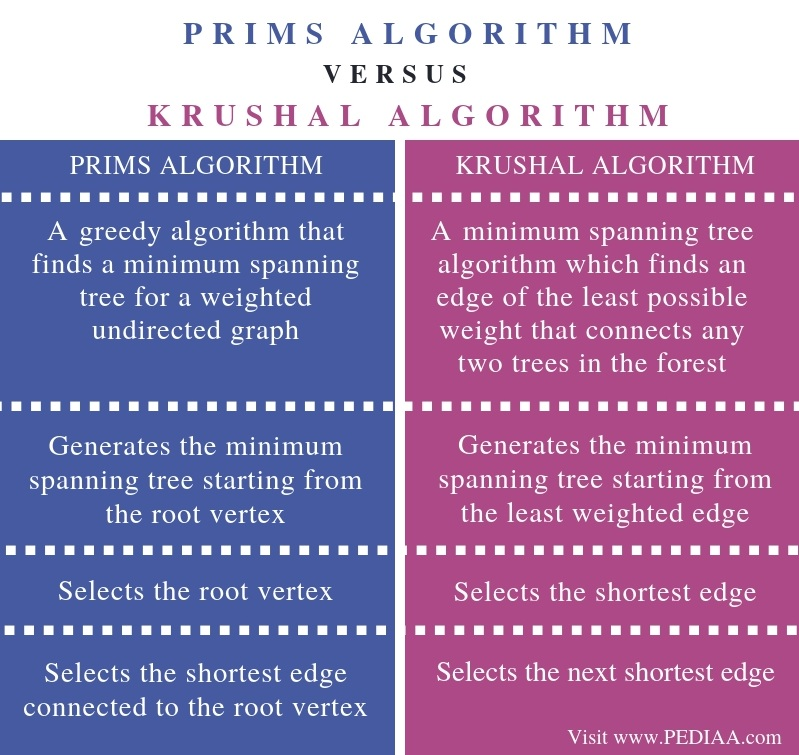# What is the Difference Between Prims and Krushal Algorithm

The main difference between Prims and Krushal algorithm is that the Prim’s algorithm generates the minimum spanning tree starting from the root vertex while the Krushal’s algorithm generates the minimum spanning tree starting from the least weighted edge.

An algorithm is a sequence of steps to follow in order to solve a problem. In greedy algorithms, we can make decisions from the given solution domain. It finds the localized optimum solution and can lead to finding globally optimized solutions. Prim’s and Krushal’s algorithms are two greedy algorithms. When there is an undirected and connected graph (G), a spanning tree is a tree that spans and is a subgraph of G. Minimum spanning tree is a spanning tree that has the minimum cost among all the spanning trees. It is mainly used in network designing. These two algorithms help to find the minimum spanning tree.

### Key Areas Covered

1. What is Prims Algorithm
– Definition, Functionality
2. What is Krushal Algorithm
– Definition, Functionality
3. What is the Difference Between Prims and Krushal Algorithm
– Comparison of Key Differences

### Key Terms

Graph, Krushal’s Algorithm, Prim’s Algorithm, Tree## What is Prims Algorithm

Prim’s algorithm helps to find the minimum spanning tree from a graph. It determines the subset of edges that include every vertex of the graph. It also reduces the sums of the weights of the edges. Also, this algorithm begins with the root node and checks all the adjacent nodes including all the connecting edges at each step. Moreover, it selects the edges with fewer weights that cause no cycles.The steps of the algorithm are as follows.

Step 1 – Select a starting vertex or a root vertex

Step 2 – Repeat step 3 and 4 until there are fringe vertices

Step 3 – Select an edge connecting the tree vertex and fringe vertex that has a minimum weight

Step 4 – Add the selected edge and the vertex to the minimum spanning tree

## What is Krushal Algorithm

Krushal’s Algorithm helps to find the minimum spanning tree for a connected weighted graph. It finds the subset of edges that can traverse every vertex of the graph. This algorithm finds an optimum solution at every stage rather than finding a global optimum.The steps of the algorithms are as follows.

Step 1- Create a forest. Each graph of the forest is a separate tree. A forest is a collection of disjoined tress. We can obtain it by deleting the root node and the edges which connect root nodes to the first level node.

Step 2- Create a priority queue that consists of all the edges of the graph.

Step 3 – Repeat step 4 and 5, if the priority queue is not empty.

Step 4 – Remove an edge from the priority queue.

Step 5 – If the resulting edge of step 4 connects two trees, then add it to the forest; if not discard the edge.

## Difference Between Prims and Krushal Algorithm

### Definition

Prim’s algorithm is a greedy algorithm that finds a minimum spanning tree for a weighted undirected graph while Krushal’s algorithm is a minimum spanning tree algorithm which finds an edge of the least possible weight that connects any two trees in the forest. This is the main difference between Prims and Krushal algorithm.

### Generation

Moreover, Prim’s algorithm generates the minimum spanning tree starting from the root vertex. However, Krushal’s algorithm generates the minimum spanning tree starting from the least weighted edge.

### Selection

Another difference between Prims and Krushal algorithm is that the Prim’s algorithm selects the root vertex whereas the Krushal’s algorithm selects the shortest edge.

### Procedure

While Prim’s algorithm selects the shortest edge connected to the root vertex, Krushal’s algorithm selects the next shortest edge. This is another difference between Prims and Krushal algorithm.

### Conclusion

Prim’s and Krushal algorithms help to find the minimum spanning tree from a graph. The difference between Prims and Krushal algorithm is that the Prim’s algorithm generates the minimum spanning tree starting from the root vertex while Krushal’s algorithm generates the minimum spanning tree starting from the least weighted edge.

##### Reference:

1. “Prim’s Algorithm – Javatpoint.” Www.javatpoint.com, Available here.
2. “Kruskal’s Algorithm – Javatpoint.” Www.javatpoint.com, Available here.
3. “Tree – Javatpoint.” Www.javatpoint.com, Available here.
4. “Prim’s Algorithm.” Wikipedia, Wikimedia Foundation, 18 Nov. 2018, Available here.
5. “Kruskal’s Algorithm.” Wikipedia, Wikimedia Foundation, 12 Dec. 2018, Available here.

##### Image Courtesy:

1. “Prim Algorithm 3” By Alexander Drichel, Stefan Birkner – Own work (CC BY-SA 3.0) via Commons Wikimedia
2. “MST kruskal en” By Schulllz – Own work (CC BY-SA 3.0) via Commons Wikimedia